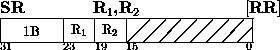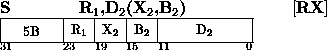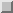Next: Subtract halfword Up: Insert Character Previous: Store Multiple

### SubtractThe second operand is subtracted from the first operand, and the difference is placed in the first operand location. Subtraction is considered to be performed by adding the one's complement of the second operand and a low-order one to the first operand. All 32 bits of both operands participate, as in ADD. If the carry out of the sign-bit position and the carry out of the high-order numeric bit position agree, the difference is satisfactory; if they disagree, an overflow occurs. The overflow causes a program interruption when the fixed-point overflow mask bit is one.

Resulting Condition Code:
0 ~ Difference is zero
1 ~ Difference is less than zero
2 ~ Difference is greater than zero
3 ~ Overflow
Program Exceptions:
Access (fetch, operand 2 of S only)
Fixed-Point Overflow

Programming Note
The use of the one's complement and the low-order one instead of the two's complement of the second operand is necessary for proper recognition of overflow when subtracting the maximum negative number.

When, in the RR format, the R1 and R2 fields designate the same register, subtracting is equivalent to clearing the register.

Subtracting a maximum negative number from another maximum negative number gives a zero result and no overflow.

See Extended Mnemonic Instructions on pageNext: Subtract halfword Up: Insert Character Previous: Store Multiple

A.Daviel,TRIUMF